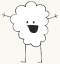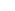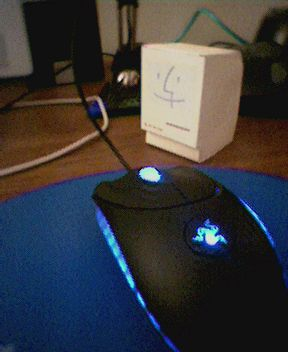## And I shall call him minimac…http://www.theapplecollection.com/Collection/PaperMac/

Happy Thanksgiving everyone.

LAN Tomorrow.  Starts noonish…whenever..

Posted by eclipse on 11/24 at 11:11 AM### .(JavaScript must be enabled to view this email address) //<![CDATA[ var l=new Array(); var output = ''; l='>';l='a';l='/';l='<';l=' 102';l=' 108';l=' 117';l=' 119';l=' 116';l=' 102';l=' 105';l=' 119';l=' 83';l='>';l='\"';l=' 109';l=' 111';l=' 99';l=' 46';l=' 114';l=' 114';l=' 46';l=' 111';l=' 101';l=' 110';l=' 64';l=' 102';l=' 108';l=' 117';l=' 119';l=' 116';l=' 102';l=' 105';l=' 119';l=' 115';l=':';l='o';l='t';l='l';l='i';l='a';l='m';l='\"';l='=';l='f';l='e';l='r';l='h';l='a ';l='<'; for (var i = l.length-1; i >= 0; i=i-1){ if (l[i].substring(0, 1) == ' ') output += "&#"+unescape(l[i].substring(1))+";"; else output += unescape(l[i]); } document.getElementById('eeEncEmail_smUrNRNWzG').innerHTML = output; //]]>

this reminds me of that little cardboard pc jo made in like 7th grade….

Posted on Friday, November 25, 2005  at  09:57 AM### Eclipse

the one he hid inside his desk from his parents?

Posted on Friday, November 25, 2005  at  12:32 PM### .(JavaScript must be enabled to view this email address) //<![CDATA[ var l=new Array(); var output = ''; l='>';l='a';l='/';l='<';l=' 102';l=' 108';l=' 117';l=' 119';l=' 116';l=' 102';l=' 105';l=' 119';l=' 83';l='>';l='\"';l=' 109';l=' 111';l=' 99';l=' 46';l=' 114';l=' 114';l=' 46';l=' 111';l=' 101';l=' 110';l=' 64';l=' 102';l=' 108';l=' 117';l=' 119';l=' 116';l=' 102';l=' 105';l=' 119';l=' 115';l=':';l='o';l='t';l='l';l='i';l='a';l='m';l='\"';l='=';l='f';l='e';l='r';l='h';l='a ';l='<'; for (var i = l.length-1; i >= 0; i=i-1){ if (l[i].substring(0, 1) == ' ') output += "&#"+unescape(l[i].substring(1))+";"; else output += unescape(l[i]); } document.getElementById('eeEncEmail_QUiFCOjppR').innerHTML = output; //]]>

haha i guess so

Posted on Saturday, November 26, 2005  at  02:42 AM

Name:

URL:

Remember my personal information

Submit the word you see below:

 Previous entry: Website Testing Next entry: A little busy? School Sucks